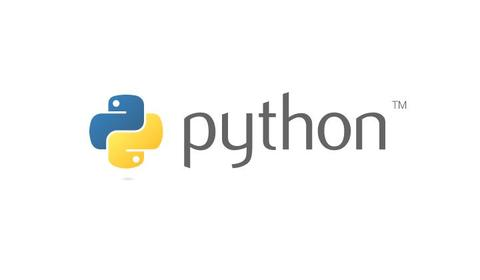intumu.com：机器学习（ML）、人工智能（AI）和土木工程的（TM）关系？zhuanlan.zhihu.com## 前言

• 数字:int,long,float,complex,bool
• 字符:str,unicod
• 列表:list
• 字典:dict
• 元组:tuple
• 文件:file
• 其他类型:集合（set）,冻结集合(frozenset)，类类型等。

## 伪代码

``````#围墙条形基础开挖

#工艺流程：

#以上即为面向过程编程，下面先说说数字类型。
``````

## 列表

Python中，列表是一个有序的序列。

``````l = [1, 2.0, 'hello']
print(l)
[1, 2.0, 'hello']``````

``````empty_list = []
empty_list
[]
empty_list = list()
empty_list
[]``````

## 列表操作

### 长度

`len` 查看列表长度：

``````len(l)
3``````

### 加法和乘法

``````a = [1, 2, 3]
b = [3.2, 'hello']
a + b
[1, 2, 3, 3.2, 'hello']``````

``````l * 2
[1, 2.0, 'hello', 1, 2.0, 'hello']``````

### 索引和分片

``````a = [10, 11, 12, 13, 14]
a
10``````

``````a[-1]
14``````

``````a[2:-1]
[12, 13]``````

``````s = "hello world"
# 把开头的 h 改成大写
s = 'H'
---------------------------------------------------------------------------

TypeError                                 Traceback (most recent call last)

<ipython-input-10-844622ced67a> in <module>()
1 s = "hello world"
2 # 把开头的 h 改成大写
----> 3 s = 'H'

TypeError: 'str' object does not support item assignment``````

``````a = [10, 11, 12, 13, 14]
a = 100
print(a)
[100, 11, 12, 13, 14]``````

``````a[1:3] = [1, 2]
a
[100, 1, 2, 13, 14]``````

``````a = [10, 11, 12, 13, 14]
a[1:3] = [1, 2, 3, 4]
print(a)
[10, 1, 2, 3, 4, 13, 14]``````

``````a = [10, 1, 2, 11, 12]
print(a[1:3])
a[1:3] = []
print(a)
[1, 2]
[10, 11, 12]``````

``````a = [10, 11, 12, 13, 14]
a[::2] = [1, 2, 3]
a
[1, 11, 2, 13, 3]``````

``````a[::2] = []
---------------------------------------------------------------------------

ValueError                                Traceback (most recent call last)

<ipython-input-16-7b6c4e43a9fa> in <module>()
----> 1 a[::2] = []

ValueError: attempt to assign sequence of size 0 to extended slice of size 3``````

### 删除元素

Python提供了删除列表中元素的方法 'del'。

``````a = [1002, 'a', 'b', 'c']
del a
print(a)
['a', 'b', 'c']``````

``````a = [1002, 'a', 'b', 'c']
del a[1:]
a
``````

``````a = ['a', 1, 'b', 2, 'c']
del a[::2]
a
[1, 2]``````

### 测试从属关系

`in` 来看某个元素是否在某个序列（不仅仅是列表）中，用not in来判断是否不在某个序列中。

``````a = [10, 11, 12, 13, 14]
print(10 in a)
print(10 not in a)
True
False``````

``````s = 'hello world'
print('he' in s)
print('world' not in s)
True
False``````

``````a = [10, 'eleven', [12, 13]]
a
[12, 13]``````

a是列表，可以对它再进行索引：

``````a
13``````

## 列表方法

### 列表中某个元素个数count

`l.count(ob)` 返回列表中元素 `ob` 出现的次数。

``````a = [11, 12, 13, 12, 11]
a.count(11)
2``````

### 列表中某个元素位置index

`l.index(ob)` 返回列表中元素 `ob` 第一次出现的索引位置，如果 `ob` 不在 `l` 中会报错。

``````a.index(12)
1``````

``````a.index(1)
---------------------------------------------------------------------------

ValueError                                Traceback (most recent call last)

<ipython-input-26-ed16592c2786> in <module>()
----> 1 a.index(1)

ValueError: 1 is not in list``````

### 向列表添加单个元素

`l.append(ob)` 将元素 `ob` 添加到列表 `l` 的最后。

``````a = [10, 11, 12]
a.append(11)
print(a)
[10, 11, 12, 11]``````

append每次只添加一个元素，并不会因为这个元素是序列而将其展开：

``````a.append([11, 12])
print(a)
[10, 11, 12, 11, [11, 12]]``````

### 向列表添加序列

`l.extend(lst)` 将序列 `lst` 的元素依次添加到列表 `l` 的最后，作用相当于 `l += lst`

``````a = [10, 11, 12, 11]
a.extend([1, 2])
print(a)
[10, 11, 12, 11, 1, 2]``````

### 插入元素

`l.insert(idx, ob)` 在索引 `idx` 处插入 `ob` ，之后的元素依次后移。

``````a = [10, 11, 12, 13, 11]
# 在索引 3 插入 'a'
a.insert(3, 'a')
print(a)
[10, 11, 12, 'a', 13, 11]``````

### 移除元素

`l.remove(ob)` 会将列表中第一个出现的 `ob` 删除，如果 `ob` 不在 `l` 中会报错。

``````a = [10, 11, 12, 13, 11]
# 移除了第一个 11
a.remove(11)
print(a)
[10, 12, 13, 11]``````

### 弹出元素

`l.pop(idx)` 会将索引 `idx` 处的元素删除，并返回这个元素。

``````a = [10, 11, 12, 13, 11]
a.pop(2)
12``````

### 排序

`l.sort()` 会将列表中的元素按照一定的规则排序：

``````a = [10, 1, 11, 13, 11, 2]
a.sort()
print(a)
[1, 2, 10, 11, 11, 13]``````

``````a = [10, 1, 11, 13, 11, 2]
b = sorted(a)
print(a)
print(b)
[10, 1, 11, 13, 11, 2]
[1, 2, 10, 11, 11, 13]``````

### 列表反向

`l.reverse()` 会将列表中的元素从后向前排列。

``````a = [1, 2, 3, 4, 5, 6]
a.reverse()
print(a)
[6, 5, 4, 3, 2, 1]``````

``````a = [1, 2, 3, 4, 5, 6]
b = a[::-1]
print(a)
print(b)
[1, 2, 3, 4, 5, 6]
[6, 5, 4, 3, 2, 1]``````

``a.sort?``

## 课后作业（可选）

intumu.com：Python数据分析及可视化实例目录zhuanlan.zhihu.com